Students can download 10th Science Chapter 3 Thermal Physics Questions and Answers, Notes, Samacheer Kalvi 10th Science Guide Pdf helps you to revise the complete Tamilnadu State Board New Syllabus, helps students complete homework assignments and to score high marks in board exams.

## Tamilnadu Samacheer Kalvi 10th Science Solutions Chapter 3 Thermal Physics

### Samacheer Kalvi 10th Science Thermal Physics Text Book Back Questions and Answers

Question 1.
The value of universal gas constant:
(a) 3.81 mol-1 K-1
(b) 8.03 mol-1 K-1
(c) 1.38 mol-1 K-1
(d) 8.31 mol-1 K-1
(d) 8.31 mol-1 K-1

Question 2.
If a substance is heated or cooled, the change in mass of that substance is:
(a) positive
(b) negative
(c) zero
(d) none of the above
(b) negative

Question 3.
If a substance is heated or cooled, the linear expansion occurs along the axis of ______.
(a) X or -X
(b) Y or -Y
(c) both (a) and (b)
(d) either (a) or (b).
(c) both (a) and (b)
Hint: When a substance is heated its expansion is positive i,e, can be taken along either +X or +Y direction. But when substance is cooled it’s either length or area or volume decreases i.e. with respect expansion, it is opposite direction i.e. either -X or -Y direction respectively.Question 4.
Temperature is the average of the molecules of a substance.
(a) difference in K.E and P.E
(b) sum of P.E and K.E
(c) difference in T.E and P.E
(d) difference in K.E and T.E
(b) sum of P.E and K.E

Question 5.
In the Given diagram, the possible direction of heat energy transformation is:(a) A ← B, A ← C, B ← C
(b) A → B, A → C, B → C
(c) A → B, A ← C, B → C
(d) A ← B, A → C, B ← C
(a) A ← B, A ← C, B ← C

II. Fill in the blanks.

1. The value of Avogadro number ………..
2. The temperature and heat are ……….. quantities.
3. One calorie is the amount of heat energy required to raise the temperature of ……….. of water through
4. According to Boyle’s law, the shape of the graph between pressure and reciprocal of volume is …………

1. 6.023 × 1023
2. Inter convertible
3. 1 gram, 1°C
4. A straight line

III. State whether the following statements are true or false, if false explain why?

1. For a given heat in liquid, the apparent expansion is more than that of real expansion.
2. Thermal energy always flows from a system at higher temperature to a system at lower temperature.
3. According to Charles’s law, at constant pressure, the temperature is inversely proportional to volume.

1. True
2. True
3. False – According to Charles law, at constant pressure, the volume is directly proportional to temperature.

IV. Match the items in column-I to the items in column-II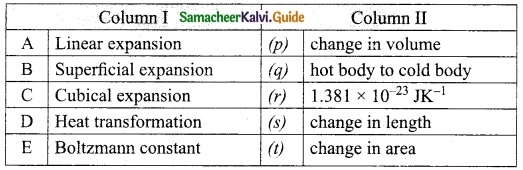A. (s)
B. (t)
C. (p)
D. (q)
E. (r)V. Assertion and Reason type questions.

(a) Both the assertion and the reason are true and the reason is the correct explanation of the assertion.
(b) Both the assertion and the reason are true but the reason is not the correct explanation of the assertion.
(c) The assertion is true but the reason is false.
(d) The assertion is false but the reason is true.
1. Assertion: There is no effects on other end when one end of the rod is only heated.
Reason: Heat always flows from a region of lower temperature to higher temperature of the rod.

2. Assertion: Gas is highly compressible than solid and liquid
Reason: Interatomic or intermolecular distance in the gas is comparably high.
1. (b)
2. (a)

Question 1.
Define one calorie.
One calorie is defined as the amount of heat energy required to rise the temperature of 1 gram of water through 1°C.

Question 2.
Distinguish between linear and superficial areal expansion.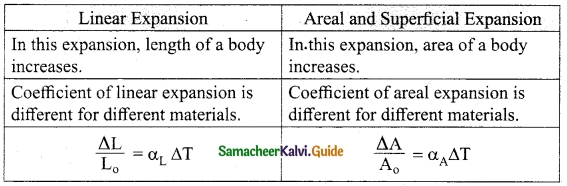Question 3.
What is the coefficient of cubical expansion?
The ratio of increase in the volume of the body per degree rise in temperature to its unit volume is called a coefficient of cubical expansion.

Question 4.
State Boyle’s law
When the temperature of a gas is kept constant, the volume of a fixed mass of gas is inversely proportional to its pressure.
P ∝ 1 / V

Question 5.
State-the law of volume.
When the pressure of a gas is kept constant, the volume of a gas is directly proportional to the temperature of the gas.
i.e., V ∝ T.
(or)
$$\frac{\mathrm{V}}{\mathrm{T}}$$ = constant.Question 6.
Distinguish between ideal gas and real gas.Question 7.
What is co-efficient of real expansion?
Coefficient of real expansion is defined as the ratio of the true rise in the volume of the liquid per degree rise in temperature to its unit volume. The SI unit of coefficient of real expansion is the K-1.

Question 8.
What is the coefficient of apparent expansion?
Coefficient of apparent expansion is defined as the ratio of the apparent rise in the volume of the liquid per degree rise in temperature to its unit volume.
The SI unit of the coefficient of apparent expansion is K-1.

VII. Numerical problems.

Question 1.
Find the final temperature of a copper rod whose area of cross section changes from 10 m² to 11 m² due to heating. The copper rod is initially kept at 90 K. (Coefficient of superficial expansion is 0.0021 /K).
Change in area ΔA = 11 – 10 = 1 m²
Initial temperature T1 = 90 K
Let Final temperature be T2K
A0 = 10 m²
Coefficient of superficial expansion is
αA = 0.0021 / k
$$\frac{ΔA}{A_0}$$ = αAΔT
$$\frac{1}{10}$$ = 0.0021 ΔT
∴ ΔT = 0.0021 × 10
= 0.021
T2 – T1 = 0.021
T2 – 90 = 0.021
∴ Final temperature T2 = 90.021 K

Question 2.
Calculate the coefficient of cubical expansion of a zinc bar. Whose volume is increased 0.25 m³ from 0.3 m³ due to the change in its temperature of 50 K.
Initial volume V0 = 0.25 m³
Final volume = 0.30 m³
Change in volume ΔV = 0.3 – 0.25 = 0.05 m³
Temperature ΔT = 50K
Coefficient of cubical expansion is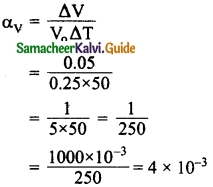∴ Coefficient of Cubical expansion
αv = 0.004 /KQuestion 1.
Derive the ideal gas equation.
The ideal gas equation is an equation, which relates all the properties of an ideal gas. An ideal gas obeys Boyle’s law and Charles’s law and Avogadro’s law.
According to Boyle’s law, PV = constant ………. (1)
According to Charles’s law,
$$\frac{V}{T}$$ = constant ……… (2)
$$\frac{V}{T}$$ = constant …….. (3)
After combining equations (1), (2) and (3), you equation. can get the following
$$\frac{V}{nT}$$ = constant ……. (4)
The above relation is called the combined law of gases. If you consider a gas, which contains µ moles of the gas, the number of atoms contained will be equal to µ times the Avogadro number, N0.
i.e., n = µNA
Using equation (5), in equation (4) can be written as
$$\frac{PV}{µN_{A}T}$$ = constant
The value of the constant in the above equation is taken to be KB, which is called as Boltzmann constant (1.38 × 10-23 JK-1). Hence, we have the following equation:
$$\frac{PV}{µN_{A}T}$$ = KB
PV = µNAKBT
µNAKB = R
which is termed as universal gas constant whose value is 8.31 J mol-1 K-1.
PV = RT
Ideal gas equation is also called as equation of state because it gives the relation between the state variables and it is used to describe the state of any gas.

Question 2.
Explain the experiment of measuring the real and apparent expansion of a liquid with a neat diagram.To start with, the liquid whose real and apparent expansion is to be determined is poured in a container up to a level. Mark this level as L1. Now, heat the container and the liquid using a burner. Initially, the container receives the thermal energy and it expands. As a result, the volume of the liquid appears to have reduced. Mark this reduced level of liquid as L2. On further heating, the thermal energy supplied to the liquid through the container results in the expansion of the liquid. Hence, the level of liquid rises to L3. Now, the difference between the levels L1 and L3 is called as apparent expansion, and the difference between the levels L2 and L3 is called real expansion. The real expansion is always more than that of apparent expansion.
Real expansion = L3 – L2
Apparent expansion = L3 – L1

IX. HOT Question

Question 1.
If you keep ice at 0°C and water at 0°C in either of your hands, in which hand you will feel more chillness? Why?
The hand consisting of ice at 0°C would feel more chillness because, ice undergoes melting. More amount of energy (chillness) is transferred to hand. In addition ice has latent heat of fusion.### Samacheer Kalvi 10th Science Thermal Physics Additional Important Questions and Answers

Question 1.
The commonly used scales of temperature are:
(a) Kelvin
(b) Celsius
(c) Fahrenheit
(d) All the above
(d) All the above

Question 2.
Ideal gas equation for n mole of gas ____.
(a) PT = nRV
(b) Pv = nRT
(c) PV = nRT
(d) PT = RV.
(b) Pv = nRT
Hint: T represents absolute temperature by t temperature in 0°C.

Question 3.
The value of 27° C in the kelvin scale:
(a) 30 K
(b) 300 K
(c) 327 K
(d) 0 K
(b) 300 K

Question 4.
Kelvin scale has zero reading at temperature _____.
(a) 0°C
(b) -100°C
(c) -273°C
(d) -212°C.
(c) -273°C
Hint: K = C + 273 or C = K – 273
at K = 0, C = -273°.Question 5.
The relation between Celsius and kelvin scales of temperature is:
(a) K = 273 – C
(b) K = C + 273
(c) K=
(d) K = C
(b) K = C + 273

Question 6.
Linear expansion is related to _____.
(a) area
(b) length
(c) volume
(d) mass.
(b) length
Hint: Linear expansion is directly proportional to the original length of rod and rise in temperature.

Question 7.
For any exchange of heat:
(a) Heat gained = Zero
(b) Heat lost = Zero
(c) Heat gained = Heat lost
(d) Heat gained = -heat lost
(c) Heat gained = Heat lost

Question 8.
………. is the degree of hotness.
(a) Heat
(b) Calorie
(c) Joule
(d) Temperature
(d) Temperature

Question 9.
(a) 6.023 × 1023
(b) 6.025 × 1025
(c) 6.24 × 1024
(d) 6.022 × 1022.
(a) 6.023 × 1023
Hint: NA = 6.023 × 1023

Question 10.
If a temperature of 327°C is equivalent to ………. in kelvin scale.
(a) 273 K
(b) 600 K
(c) -527 K
(d) -273 K
(b) 600 K

Question 11.
When spirit is poured on our hand, cooling is produced because:
(a) Spirit has cooling effect.
(b) Spirit has boiling effect.
(c) The boiling point of spirit is low.
(d) The boiling point of spirit is high.
(c) The boiling point of spirit is low.

Question 12.
Process of transfer of heat through liquid and gases is _____.
(a) conduction
(c) convection
(d) none of these.
(c) convection
Hint: Heat flows by the conventional current is upward direction by convection method.

Question 13.
Heat required to melt 1 kg of ice at 0°C is:
(a) 226 × 102 J
(b) 336 × 103 J
(c) 353 × 103 J
(d) 3 × 105 J
(b) 336 × 103 J

Question 14.
Relation between α, β and γ is _____.
(a) α = β = γ
(b) $$\alpha=\frac{\beta}{2}=3 \gamma$$
(c) $$\alpha=\frac{\beta}{2}=\frac{\gamma}{3}$$
(d) $$\alpha=\frac{\beta}{2}=\frac{\gamma}{4}$$.
(c) $$\alpha=\frac{\beta}{2}=\frac{\gamma}{3}$$
Hint: (c) $$\alpha=\frac{\beta}{2}=\frac{\gamma}{3}$$ (or) 6α = 3β = 2γ.Question 15.
When a certain quantity of ice is melting remains the same.
(a) Volume
(b) Temperature
(c) Mass
(d) Density
(b) Temperature

Question 16.
Steam causes more severe burns than water at the same temperature because steam:
(a) is in vapour state
(b) contains less heat than water at the same temperature.
(c) contains more heat than water at the same temperature.
(d) cause bums by nature.
(c) contains more heat than water at the same temperature.

Question 17.
Which expansion coefficient (α, β, γ) of a substance has the largest and y smallest magnitude?
(a) α, β
(b) α, γ
(c) γ, α
(d) β, α.
(c) γ, α
Hint: As γ is 3 times of α and β is 2 times of α. so α is minimum and γ is maximum.

Question 18.
According to the principle of mixtures, the heat lost by a hot body is equal to:
(a) Heat gained by the surroundings
(b) Heat transferred to the surroundings
(c) Heat gained by the body
(d) None of the above
(c) Heat gained by the body

Question 19.
The quantity of water vapour required to saturate air at high temperature is:
(a) Less
(b) Temperature
(c) More
(d) None of the above
(c) More

Question 20.
In steam heater, solids attain constant temperature because:
(a) Solid should not be heated less
(b) Solid should not be heated more
(c) Melting point of solid is 100°C
(d) Volume does not change.
(c) Melting point of solid is 100°C

Question 21.
The quantity of water vapour required to saturate air depends on:
(a) Pressure of atmosphere
(b) Temperature of atmosphere
(c) Humidity of atmosphere E
(d) All the above
(b) Temperature of atmosphere

Question 22.
Volume of a gas at t°C is given by: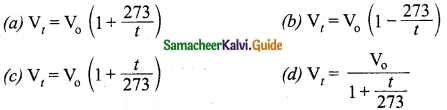(c) Vt = Vo (1 + $$\frac{t}{273}$$)

Question 23.
At a higher temperature to saturate air, ………. quantity of water vapour is required.
(a) Less
(b) Some
(c) More
(d) No
(c) More

Question 24.
The relationship between length (L0) of a body and change in temperature is: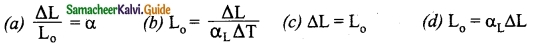(b) L0 = $$\frac{ΔL}{α_{L}ΔT}$$

Question 25.
The S.l unit of coefficient of linear expansion is:
(a) °C
(b) K-1
(c) Cal
(d) Joule
(b) K-1Question 26.
Coefficient of superficial expansion:
(a) is same for all materials
(b) is infinity
(c) different for different materials
(d) is zero
(c) different for different materials

Question 27.
The ratio of change in area of a metal to its original area is $$\frac{ΔA}{A_0}$$ =
(a) αA
(b) αAΔT
(c) $$\frac{α_A}{ΔT}$$
(d) unity
(b) αAΔT

Question 28.
According to Boyle’s law the relation between pressure (P) and volume of a gas is:
(a) P ∝ V
(b) P = V
(c) P ∝$$\frac{1}{V}$$
(d) V ∝ P
(c) P ∝$$\frac{1}{V}$$

Question 29.
At constant temperature of a gas:
(a) PV = 1
(b) PV = 0
(c) PV = infinity
(d) PV = constant
(d) PV = constant

Question 30.
The mathematical form of Charles’s law is:
(a) V ∝ $$\frac{1}{T}$$
(b) TV = constant
(c) $$\frac{V}{T}$$ = constant
(d) V = T
(c) $$\frac{V}{T}$$ = constant

Question 31.
If V is the volume and n is the number of atoms present in it then:
(a) V ∝ $$\frac{1}{n}$$
(b) V ∝ n
(c) V = n
(d) $$\frac{n}{V}$$ = constant
(b) V ∝ n

Question 32.
$$\frac{V}{n}$$ = constant is the mathematical form of:
(a) Boyle’s law
(b) Charles’s law
(d) Dalton’s law

Question 33.
Mathematical form of Boyle’s law is:
(a) $$\frac{V}{n}$$ = constant
(b) PT = constant
(c) $$\frac{V}{T}$$ = constant
(d) PV = constant
(d) PV = constant

Question 34.
A gas that obeys Boyle’s law and Charles’s law is called:
(a) Gas
(b) Ideal gas
(c) Perfect gas
(d) All the above
(b) Ideal gas

Question 35.
The value of universal gas constant is:
(a) 3.81 J/mol/K
(b) 8.31 J/mol/K
(c) 8.13
(d) 6.81 J/mol/K
(b) 8.31 J/mol/KQuestion 36.
The unit of universal gas constant is:
(a) $$\frac{J}{K}$$
(b) J mol-1K
(c) J/mol/K
(d) J K-1 mol
(c) J/mol/K

Question 37.
If atoms of a gas do not interact with each other than the gas is:
(a) natural gas
(b) bio gas
(c) real gas
(d) perfect gas
(d) perfect gas

Question 38.
Mathematical form of ideal gas equation is:
(a) PV = T
(b) P = RT
(c) PV = RT
(d) PV = R
(c) PV = RT

II. Fill in the blanks.

1. The value if 290K in Celsius scale is ……….
2. The value of 37°C in kelvin scale is ……….
3. The value of 323 K in Celsius scale is ……….
4. Transfer of heat is continued until a ………. is established.
5. ……….. produces the sensation of warm.
6. When a body is heated or cooled its ……….. is not altered.
7. For any exchanges of heat …………. = …………
8. On heating all forms of matter undergo ………..
9. The coefficient of linear expansion is ………. for ……….. metals.
10. The unit of coefficient of superficial expansion is …………
11. The coefficient of cubical expansion of liquid is independent of …………
12. The S.l of unit of coefficient of real expansion is ………..
13. As per Boyle’s law pressure of a gas is …………. proportional to its volume.
14. PV = constant is the mathematical form of ………….
15. As per Charles’s law volume of a gas is ………… to temperature.
16. According Avogadro’s law volume of a gas is directly proportional ………… present in it.
17. The value of Avogadro’s number is …………
18. A gas that obey Boyle’s law is …………
19. A gas that does not obey gas laws then it is …………
20. A gas in which atoms interact with a force then it is a ………..
21. For a given heat, the real expansion is ……….. than that of apparent expansion.
22. The equation of state of a gas is …………
23. Universal gas equation is used to describe the ………….
24. If a gas consists of µ moles then the number of atoms in n = …………
1. 17°C
2. 310 K
3.50°C
4. thermal equilibrium
5. Heat
6. mass
7. Heat gained, Heat lost
8. expansion
9. different, different
10. K-1
11. Temperature
12. K-1
13. inversely
14. Boyle’s law
15. directly proportional
16. number of atoms or molecules
17. 6.023 × 1023/mol
18. ideal gas
19. real gas
20. real gas
21. more
22. PV = RT
23. state of any gas
24. µNA, NA – Avogadro’s numberIII. State whether the following statements are true or false, if false explain why?

1. The relation between Fahrenheit and Kelvin scale of temperature is (K) K = (F + 460) × $$\frac{5}{9}$$.
2. The relation between Celsius and Kelvin is K = C – 273.
3. Thermal energy is also known as heat energy.
4. When a body is heated volume is not altered.
5. All forms of matter undergo expansion on heating.
6. Longitudinal expansion is given by ΔL = L0αLΔT
7. Cubical expansion is same for all materials.
8. The S.l unit of coefficient of apparent expansion is K-1.
9. As per Boyle’s law PT = constant.
10. According to Avogadro’s law $$\frac{V}{n}$$ = constant
1. True
2. False -The relation between Celsius and Kelvin is K= C + 273
3. True
4. False – When a body is heated mass is not altered.
5. True
6. True
7. False – Cubical expansion is different for different materials.
8. True
9. False – As per Boyle’s law PV= constant.
10. True

IV. Match the items in column-I to the items in column-II.

Question 1.
Match the following:A – (s)
B – (t)
C – (p)
D – (q)

Question 2.
Match the following: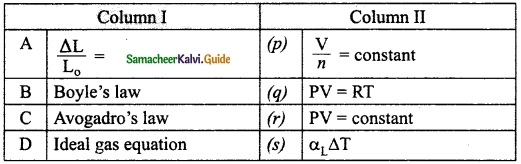A – (s)
B – (r)
C – (p)
D – (q)

Question 3.
Match the following:A – (t)
B – (s)
C – (p)
D – (q)

V. Assertion and reason type questions.

Question 1.
Assertion: In a pressure cooker, the water starts boiling again on removing its lid.
Reason: The impurities in water bring down its boiling point.
(a) Both the assertion and the reason are true and the reason is the correct explanation of the assertion.
(b) Both the assertion and the reason are true but the reason is not the ’ correct explanation of the assertion.
(c) The assertion is true but the reason is false.
(d) The assertion is false but the reason is true.
(c) The assertion is true but the reason is false.

Question 2.
Assertion: Air at some distance above the fire is hotter than same distance below it.
Reason: Air surrounding the fire carries heat upwards.
(a) Both the assertion and the reason are true and the reason is the correct explanation of the assertion.
(b) Both the assertion and the reason are true but the reason is not the correct explanation of the assertion.
(c) The assertion is true but the reason is false.
(d) The assertion is false but the reason is true.
(a) Both the assertion and the reason are true and the reason is the correct explanation of the assertion.Question 3.
Assertion: Woolen clothes keys the body warm in winter.
Reason: Air a poor conducts of heat.
(a) Both the assertion and the reason are true and the reason is the correct explanation of the assertion.
(b) Both the assertion and the reason are true but the reason is not the correct explanation of the assertion.
(c) The assertion is true but the reason is false.
(d) The assertion is false but the reason is true.
(a) Both the assertion and the reason are true and the reason is the correct explanation of the assertion.

Question 4.
Assertion: Temperature near the sea coast is moderate.
Reason: Water has a high thermal conductivity.
(a) Both the assertion and the reason are true and the reason is the correct explanation of the assertion.
(b) Both the assertion and the reason are true but the reason is not the correct explanation of the assertion.
(c) The assertion is true but the reason is false.
(d) The assertion is false but the reason is true.
(b) Both the assertion and the reason are true but the reason is not the correct explanation of the assertion.

Question 5.
Assertion: It is hotter over the top of fire than at the same distance on the sides.
Reason: Air surrounding the fire conducts more heat upwards.
(a) Both the assertion and the reason are true and the reason is the correct explanation of the assertion.
(b) Both the assertion and the reason are true but the reason is not the correct explanation of the assertion.
(c) The assertion is true but the reason is false.
(d) The assertion is false but the reason is true.
(c) The assertion is true but the reason is false.

Question 6.
Assertion: Perspiration from human body helps in cooling the body. Reason: A thin layer of water on the skin enhance its emissivity.
(a) Both the assertion and the reason are true and the reason is the correct explanation of the assertion.
(b) Both the assertion and the reason are true but the reason is not the correct explanation of the assertion.
(c) The assertion is true but the reason is false.
(d) The assertion is false but the reason is true.
(c) The assertion is true but the reason is false.

Question 1.
Define Temperature.
Temperature is defined as the property which determines whether a body is in equilibrium or not with the surroundings.

Question 2.
Why the gas thermometer is more sensitive than Hg thermometer
As the thermal (cubical) expansion of gas is much larger than Hg. So gas thermometer is more sensitive than of Hg thermometer.

Question 3.
What is meant by thermodynamic temperature?
The temperature measured in relation to absolute zero using the kelvin scale is known as absolute temperature. It is also known as the thermodynamic temperature.

Question 4.
What is the relation between different types of scale of temperature?
The relation between the different types of scale of temperature:
Celsius and Kelvin: K = C + 273,
Fahrenheit and Kelvin: [K] = (F + 460) × $$\frac{5}{9}$$.
0 K = -273°C.Question 5.
Do all liquids expand on heating? give an example.
All liquids do not expand on heating. If water is heated from 0°C to 4°C it contracts.

Question 6.
What will happen if two bodies are at different temperatures brought in contact with one other?
There will be a transfer of heat energy from the hot body to the cold body until a thermal equilibrium is established between them.

Question 7.
What will happen if a cold body is placed in contact with a hot body?
Some thermal energy is transferred from the hot body to the cold body. As a result, there is some rise in the temperature of the cold body and decrease in the temperature of the hot body. This process will continue until these two bodies attain the same temperature.

Question 8.
Why is invar is used in making a clock pendulum or spring to oscillate?
Invar an alloy of Ni and steel has extremely low thermal expansion so the change in length in summer and winter will be a very small change, so the time period of oscillation will be very small. Hence the clock gives almost the correct time.

Question 9.
What is meant by heating?
The process in which heat energy flows from a body at a higher temperature to another body at lower temperature is known as heating.

Question 10.
What is the average velocity of the molecules of an ideal gas?
As the velocity components of molecules of an ideal gas, all three axis time and time axis are equal in magnitude so their vector sum will be zero. So every velocity of an ideal gas is zero.

Question 11.
What changes will occur when heat is given to a substance?

1. Temperature of the substance rises.
2. The substance may change its state from solid to liquid or from liquid to gas. (Hi) The substance will expand when heated.

Question 12.
Why does the temperature less than zero on the absolute scale not possible.
As the absolute temperature (T) is directly proportioned to KE of molecules of gas, and KE of molecules can never be negative so the absolute scale temperature can never be negative.

Question 13.
What is meant by linear expansion?
When a body is heated or cooled, the length of the body changes due to change in its temperature. Then the expansion is said to be linear or longitudinal expansion.

Question 14.
Write the characteristics of an ideal gas.

1. It obeys all gas laws at all values of temperature pressure.
2. Size of molecules is negligibly small.
3. There is no force of attraction or repulsion between its molecule.

Question 15.
Mention the relation between change in length and coefficient of linear expansion?
The equation relating the change in length and the change in temperature of a body is given below:
$$\frac{ΔL}{L_0}$$ = αLΔT
ΔL – Change in length (Final length – Original length)
L0 – Original length
ΔT – Change in temperature (Final temperature – Initial temperature)
αL – Coefficient of linear expansion.Question 16.
What is meant by superficial expansion?
If there is an increase in the area of a solid object due to heating, then the expansion is called superficial or areal expansion.

Question 17.
Define co-efficient of superficial expansion.
The ratio of increase in area of the body per degree rise in temperature to its unit area is called as coefficient of superficial expansion.

Question 18.
State the relation between change in area and change in temperature.
$$\frac{ΔA}{A_0}$$ = αAΔT
ΔA – Change in area (Final area – Initial area)
A0 – Original area
ΔT – Change in temperature (Final temperature – Initial temperature)
αA – Coefficient of superficial expansion.

Question 19.
What is meant by cubical expansion?
If there is an increase in the volume of a solid body due to heating, then the expansion is called cubical or volumetric expansion.

Question 20.
Write the equation relation the change in volume and the change in temperature.
$$\frac{ΔV}{V_0}$$ = αAΔT
ΔV – Change in volume (Final volume – Initial volume)
V0 – Original volume
ΔT – Change in temperature (Final temperature – Initial temperature)
αV – Coefficient of cubical expansion.

Question 21.
What is real expansion of a liquid?
If a liquid is heated directly without using any container, then the expansion that you observe is termed as real expansion of the liquid.

Question 22.
What is meant by apparent expansion of a liquid?
The expansion of a liquid when observed without considering the expansion of the container is called the apparent expansion of the liquid.

Question 23.
Avogadro’s law states that at constant pressure and temperature, the volume of a gas is directly proportional to number of atoms or molecules present in it.
i.e., V α n
(or) $$\frac{V}{n}$$ = constant

Question 24.
Avogadro’s number (NA) is the total number of atoms per mole of the substance. It is equal to 6.023 × 1023/mol.

Question 25.
What are real gases?
If the molecules or atoms of a gases interact with each other with a definite amount of intermolecular or inter atomic force of attraction, then the gases are said to be real gases.Question 26.
What is a perfect gas?
If the atoms or molecules of a gas do not interact with each other, then the gas is said to be an ideal gas or a perfect gas.

Question 27.
What is an ideal gas equation?
The ideal gas equation is an equation, which relates all the properties of an ideal gas.

Question 28.
Why is ideal gas equation called as equation of state?
Ideal gas equation is also called as equation of state because it gives the relation between the state variables and it is used to describe the state of any gas.

Question 29.
Define each unit of a thermodynamic scale of temperature.
Each unit of the thermodynamic scale of temperature is defined as the fraction of 1/273.16th part of the thermodynamic temperature of the triple point of water.

VII. Numerical problems.

Question 1.
Transform 100°C into K.
T (kelvin) = (273 + t°C) K
= (273 + 100) K
= 373 K
100°C = 373 K

Question 2.
Convert 23 K into °C.
T = 23 K
T°C = K – 273
= 23 – 273
= -250°C
23 K = -250°C

Question 3.
If the gap between steel sails on the railway track of 66 m long is 3.63 cm at 10°C. Then at what value of temperature will just touch of steel is 11 × 10-6 °C.
Solution:
L0 = 66 m = 6600 cm
α = 11 × 10-6 °C.
∆L = Lt – L0 = 3.63
t1 = 10°C
t2 = ?
$$\alpha=\frac{\Delta \mathrm{L}}{\mathrm{L}_{\mathrm{o}} \Delta \mathrm{T}}$$
$$\begin{array}{l}{\Delta \mathrm{T}=\frac{\Delta \mathrm{L}}{\mathrm{L}_{0} \times \alpha}} \\ {\Delta \mathrm{T}=\frac{3.63}{6600 \times 11 \times 10^{-6}}}\end{array}$$
∆T = t2 – t1 = 50
⇒ t2 – 10 = 50
⇒ t2 = 50 + 10 = 60°C
so final temperature t2 = 60°C

Question 4.
At what temperature do the ratings of Celsius and Fahrenheit scales coincide?
Let TB = TB – x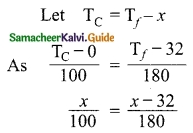∴ 180x = 100x – 3200
80x = -3200
x = –$$\frac{3200}{80}$$ = -40°
x = -40°
∴ Hence -40°C and -40° f are identical Temperature.Question 5.
On heating a glass block of 105 cm³ from 25°C to 40°C its volume increases by 4 cm³. Calculate the coefficient of
(i) Cubical expansion and
(ii) Linear expansion
Volume V0 = 105 cm³
Change in temperature ΔT = 40 – 25 = 15°C
Change in volume ΔV = 4 cm³
(i) The coefficient of cubical expansion isαV = 26.67 × 10-6/°C

(ii) Coefficient of linear expansion is
αL = $$\frac{αV}{3}$$
αL = $$\frac{26.67}{3}$$ × 10-6
αL = 8.89 × 10-6/°C

Question 6.
A balloon partially filled with the gas volume 30 m3 at on surface of the earth where pressure is 76 cm of Hg and temperature is 27°C. What will be the increase in the volume of the gas balloon when it rises to a height where the temperature becomes (-54°C) and pressure become 7.6 cm of Hg.
Solution:
Given, P1 = 76 cm Hg, P2 = 7.6 cm of Hg
V1 = 30 m3, V2 = ?
T1 = 27 + 273 = 300 K
T2 = -54 + 273 = 219 K
By gas equation
$$\frac{\mathrm{P}_{1} \mathrm{V}_{1}}{\mathrm{T}_{1}}=\frac{\mathrm{P}_{2} \mathrm{V}_{2}}{\mathrm{T}_{2}}$$
$$\mathrm{V}_{2}=\frac{\mathrm{P}_{1} \mathrm{V}_{1} \mathrm{T}_{2}}{\mathrm{T}_{1} \mathrm{P}_{2}}=\frac{76 \times 30 \times 219}{300 \times 7.6}$$ = 219 m3
So increase in volume of gas = 219 – 30 = 189 m3.

Question 7.
If the area of metal changes by 0.22% when it is heated through 10°C, then calculate the coefficient of superficial expansion.
$$\frac{ΔA}{A}$$ = 0.22% = $$\frac{0.22}{100}$$
Change in temperature ΔT = 10°C
∴ Coefficient of cubical expansion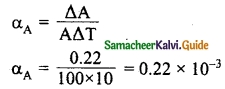= 22 × 10-6/°C

Question 8.
Using the ideal gas equation determine the value of universal gas constant. It is given that one gram, molecule of a gas at S.T.P occupies 22.4 litres.
Pressure P = 1.013 × 105 pa
Volume V = 22.4 lit I
= 22.4 × 10-3
Temperature T = 273 K
For one mole of a gas
PV = RT
∴ R = $$\frac{PV}{T}$$= 8.31 J/mol/K

Question 9.
When a gas filled in a closed vessel is heated through 1°C, its pressure increases by 0.4% what is the initial temperature of the gas?
Initial pressure P1 = P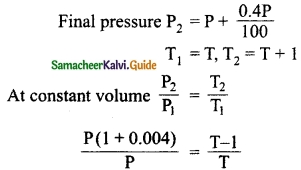PT + 0.004PT = PT + P
0.004PT = P
∴ T = $$\frac{1}{0.004}$$
= 250 K
∴ Initial Temperature of the gas = 250 K

Question 10.
A vessel of volume 2000 cm³ contains 0.1 mole of O2 and 0.2 mole of CO2 . If the temperature of the mixture is 300 K then calculate the pressure exerted by it.
n1 = 0.1; n2 = 0.2; R = 8.31 J/mol/K;
Temperature T = 300K; Volume V = 2000 × 10-6
Pressure P = P1 + P2P = 3.74 × 105 paQuestion 1.
Explain how the loss of heat (or transfer of heat) due to modes of transfer of heat is minimised in a thermos flask.
Transfer of heat is thermos is minimised as under:
(i) By conduction: As in conduction heat can transfer by contact of a material medium. In thermos, the air is evacuated between the walls so heat transfer is stopped by conduction mode.

(ii) By convection: As convection mode also requires material (fluid) medium and there is nothing between the walls of thermos so heat does not transfer by connection mode.

(iii) By Radiation: As Ag polish is coated opaque on inner and outer walls of thermos radiation obeys the laws of refraction and reflection so no refraction takes place through opaque wall.
Reflection of outer radiation goes outside of the inner wall goes inside. So the transfer of heat is minimised by polishing.

Question 2.
Explain linear expansion in Solids.
When a body is heated or cooled, the length of the body changes due to change in its temperature. Then the expansion is said to be linear or longitudinal expansion.

The ratio of increase in length of the body per degree rise in temperature to its unit length is called as the coefficient of linear expansion. The SI unit of Coefficient of Linear expansion is K-1. The value of coefficient of linear expansion is different for different materials.The equation relating the change in length and the change in temperature of a body is given below:
$$\frac{ΔL}{L_0}$$ = αLΔT
ΔL – Change in length (Final length – Original length)
L0 – Original length
ΔT – Change in temperature (Final temperature – Initial temperature)
αL – Coefficient of linear expansion.

Question 3.
Write a note on superficial expansion.
If there is an increase in the area of a solid object due to heating, then the expansion is called superficial or areal expansion.

Superficial expansion is determined in terms of coefficient of superficial expansion. The ratio of increase in area of the body per degree rise in temperature to its unit area is called as coefficient of superficial expansion. Coefficient of superficial expansion is different for different materials. The SI unit of Coefficient of superficial expansion is K-1.

The equation relating to the change in area and the change in temperature is given below:$$\frac{ΔA}{A_0}$$ = αAΔT
ΔA – Change in area (Final area – Original area)
A0 – Original area
ΔT – Change in temperature (Final temperature – Initial temperature)
αA – Coefficient of superficial expansion.

Question 4.
What do you know about cubical expansion?
If there is an increase in the volume of a solid body due to heating, then the expansion is called cubical or volumetric expansion. As in the cases of linear and areal expansion, cubical expansion is also expressed in terms of coefficient of cubical expansion. The ratio of increase in volume of the body ‘ per degree rise in temperature to its unit volume is called as coefficient of cubical expansion. This is also measured in K-1.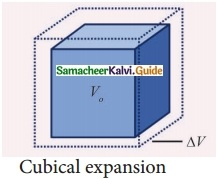The equation relating to the change in volume and the change in temperature is given below:
$$\frac{ΔV}{V_0}$$ = αVΔT
ΔV – Change in volume (Final volume – Original volume)
V0 – Original volume
ΔT – Change in temperature (Final temperature – Initial temperature)
αV – Coefficient of cubical expansion.IX. Hot Questions.

Question 1.
At what common temperature a block of wood metal appear equally cold or hot when touched?
A body appears hot when touched if heat flows from the body to our hand and vice – versa. If there is no flow of heat across the body and hand, the body can not be identified while it is hot or cold as bodies are in thermal equilibrium with our body. So both block of wood and metal must have the temperature of our body i.e., 37°C.

Question 2.
At room temperature water does not sublimate from ice to steam. Give reason.
The critical temperature of the water is much above room temperature.

Question 3.
Good conductors of heat are also good conductors of electricity and vice versa why?
It is because of the movement of electrons present in the materials.

Question 4.
When does the Charle’s law fail?
By Charle’s law at constant pressure
V ∝ T or T ∝ V
At T = 0 K volume must be zero but it is impossible, at low-temperature gases does not obey the characterise of the ideal gas. As the molecules come closer and force of attraction and repulsion takes place.

Question 5.
When sugar is added to tea it gets cooled, why?